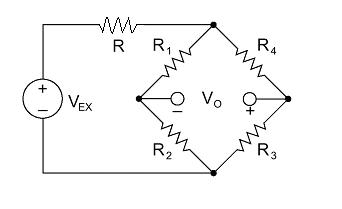# On bridgesFor the Wheatstone bridge above, let $R = \frac {391}{13}\:\Omega,\: R_2 = 252\:\Omega,\: R_4 = 120\:\Omega,\: V_{EX} = 5\: V$ dc. If $V_O = 0 \: V$, and the voltage drop across $R$ is $\frac {1955}{2743} \: V$, determine the sum (in ohms) of the resistances $R_1$ and $R_3$.

×